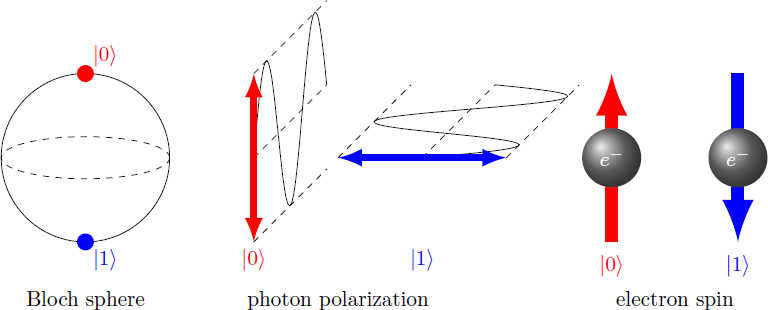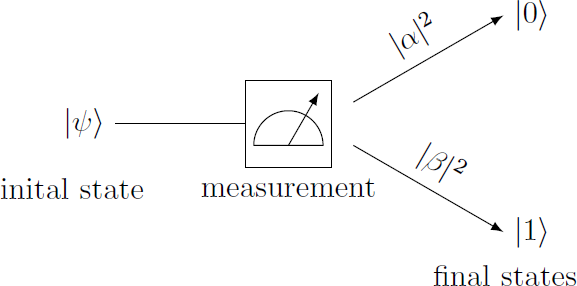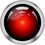# Quantum Computing 1 -- The qubitThe qubit is the smallest storage unit in the quantum computer and represents the simplest nontrivial quantum system. The qubit can assume one of two distinguishable states. These eigenstates of the qubit are denoted by $| 0 \rangle$ and $| 1 \rangle$ and correspond to the two setting possibilities of a classical bit. They can also be represented as two-dimensional unit vectors $|0\rangle = \left( \begin{array}{c} 1 \\ 0 \end{array} \right), \quad |1\rangle = \left( \begin{array}{c} 0 \\ 1 \end{array} \right)$ In contrast to the classical bit, the qubit can also assume a superposition of the states $| 0 \rangle$ and $| 1 \rangle$. The general state $| \psi \rangle$ of the qubit is therefore a linear combination of the two eigenstates $|\psi\rangle = \alpha |0\rangle + \beta |1 \rangle = \left( \begin{array}{c} \alpha \\ \beta \end{array} \right), \quad \alpha, \beta \in \mathbb{C}$ The vectors $| 0 \rangle$ and $| 1 \rangle$ thus form a basis of the vector space. The state $| \psi \rangle$ is characterized by the two complex numbers $\alpha$ and $\beta$.If the qubit is in such a superposition and you take a measurement, you get a random result. The probability of finding the qubit in the state $| 0 \rangle$ or $| 1 \rangle$ is proportional to the absolute square $| \alpha | ^ 2$ or $| \beta | ^ 2$ of the respective pre-factor. After the measurement, the qubit is in one of the eigenstates $| 0 \rangle$ or $| 1 \rangle$, so that the information about the original state is lost (wave function collapse)

\begin{aligned} \text{before measurement:} & & |\psi \rangle &= \alpha |0 \rangle + \beta |1\rangle \\ \text{after measurement:} & & |\psi'\rangle &= \begin{cases} |0 \rangle & \text{with probability } \frac{|\alpha|^2}{|\alpha|^2 + |\beta|^2} \\ |1 \rangle & \text{with probability } \frac{|\beta|^2}{|\alpha|^2 + |\beta|^2} \end{cases} \end{aligned} Usually, state vectors will be normalized (i.e. $| \alpha | ^ 2 + | \beta | ^ 2 = 1$), so the probabilities for the states $| 0 \rangle$ and $| 1 \rangle$ are given directly by absolute squares $| \alpha | ^ 2$ and $| \beta | ^ 2$. In addition, a phase factor can be chosen freely, so that the qubit can be described by two real numbers $\theta$ and $\varphi$: $|\psi\rangle = \cos \frac{\theta}{2} |0\rangle + e^{i \varphi} \sin \frac{\theta}{2}|1\rangle$ If one interprets these numbers $\theta$ and $\varphi$ as angles, the qubit can be represented as a point on a sphere surface (Bloch sphere).

A qubit in the normalized state $|\psi\rangle = \frac{\sqrt{3}}{2} |0\rangle + \frac{1}{2} |1\rangle$ is measured. What are the probabilities of the two different outcomes $| 0 \rangle$ and $| 1 \rangle$?

\begin{aligned} |0\rangle & \quad \text{ with probability } \left|\frac{\sqrt{3}}{2}\right|^2 = \frac{3}{4} = 75\,\%,\\ |1\rangle & \quad \text{ with probability } \left|\frac{1}{2}\right|^2 = \frac{1}{4} = 25\,\% \end{aligned}

Conclusion: The state of a qubit is described by a two-dimensional vector, so that a qubit contains much more information than a classical bit. However, if the qubit is read out, most information is deleted, so that the result is only one of the two states $| 0 \rangle$ or $| 1 \rangle$. The readable part of the quantum information therefore only corresponds to one classical bit. However, the full quantum information be can used for quantum operations, as we will see later.Note by Markus Michelmann
3 years, 1 month ago

This discussion board is a place to discuss our Daily Challenges and the math and science related to those challenges. Explanations are more than just a solution — they should explain the steps and thinking strategies that you used to obtain the solution. Comments should further the discussion of math and science.

When posting on Brilliant:

• Use the emojis to react to an explanation, whether you're congratulating a job well done , or just really confused .
• Ask specific questions about the challenge or the steps in somebody's explanation. Well-posed questions can add a lot to the discussion, but posting "I don't understand!" doesn't help anyone.
• Try to contribute something new to the discussion, whether it is an extension, generalization or other idea related to the challenge.

MarkdownAppears as
*italics* or _italics_ italics
**bold** or __bold__ bold
- bulleted- list
• bulleted
• list
1. numbered2. list
1. numbered
2. list
Note: you must add a full line of space before and after lists for them to show up correctly
paragraph 1paragraph 2

paragraph 1

paragraph 2

[example link](https://brilliant.org)example link
> This is a quote
This is a quote
    # I indented these lines
# 4 spaces, and now they show
# up as a code block.

print "hello world"
# I indented these lines
# 4 spaces, and now they show
# up as a code block.

print "hello world"
MathAppears as
Remember to wrap math in $$ ... $$ or $ ... $ to ensure proper formatting.
2 \times 3 $2 \times 3$
2^{34} $2^{34}$
a_{i-1} $a_{i-1}$
\frac{2}{3} $\frac{2}{3}$
\sqrt{2} $\sqrt{2}$
\sum_{i=1}^3 $\sum_{i=1}^3$
\sin \theta $\sin \theta$
\boxed{123} $\boxed{123}$

Sort by:

I hope to see more additions to the series :)

- 3 years, 1 month ago

After almost two weeks break there is non a sequel to this series with a new chapter. I hope you like it :D

- 3 years ago